Trial ends in
JoVE Science Education
Mechanical Engineering

A subscription to JoVE is required to view this content.

Determination of Impingement Forces on a Flat Plate with the Control Volume Method
##### Science Education (Mechanical Engineering)
Piping Networks and Pressure Losses
##### Science Education (Mechanical Engineering)
Stability of Floating Vessels

# Determination of Impingement Forces on a Flat Plate with the Control Volume Method

### Overview

Source: Ricardo Mejia-Alvarez and Hussam Hikmat Jabbar, Department of Mechanical Engineering, Michigan State University, East Lansing, MI

The purpose of this experiment is to demonstrate forces on bodies as the result of changes in the linear momentum of the flow around them using a control volume formulation [1, 2]. The control volume analysis focuses on the macroscopic effect of flow on engineering systems, rather than the detailed description that could be achieved with a differential analysis. Each one of these two techniques have a place in the toolbox of an engineering analyst, and they should be considered complementary rather than competing approaches. Broadly speaking, control volume analysis will give the engineer an idea of the dominant loads in a system. This will give her/him an initial feeling about what route to pursue when designing devices or structures, and should ideally be the initial step to take before pursuing any detailed design or analysis via differential formulation.

The main principle behind the control volume formulation is to replace the details of a system exposed to a fluid flow by a simplified free body diagram defined by an imaginary closed surface dubbed the control volume. This diagram should contain all surface and body forces, the net flux of linear momentum through the boundaries of the control volume, and the rate of change of linear momentum inside the control volume. This approach implies cleverly defining the control volume in ways that simplify the analysis at the same time that capture the dominant effects on the system. This technique will be demonstrated with a plane jet impinging on a flat plate at different angles. We will use control volume analysis to estimate the aerodynamic load on the plate, and will compare our results with actual measurements of the resulting force obtained with an aerodynamic balance.

### Principles

A control volume (CV) is defined by an imaginary closed surface, dubbed the control surface (CS), defined arbitrarily to study the effect of flow around objects and systems. Figure 1 shows an example of a control volume containing a region of flow going around a solid object. The flow in the immediate vicinity of the object is highly complex, and we would like to avoid that complexity in order to estimate the global effect of the flow on the supporting element. Once defined, the CV becomes a free body diagram that captures the interactions between the flow and the enclosed object that give rise to loads in the supporting system. To this end, we equate the surface and body forces on the CV with the change of linear momentum of the flow that goes through the CV. The surface forces are pressure, flow-induced shear, and any reactions of the solids "cut" by the control volume. The body forces are basically the weight of everything contained in the control volume, including solids and fluids, and any other force induced by volumetric effects such as electromagnetic fields. The change in linear momentum of the flow is the added effect of the net flux of momentum through the CS and the rate of change of momentum contained in the CV. All these effects can be summarized in the equation for conservation of linear momentum in integral form: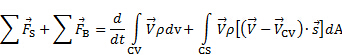(1)

Here,are the surface forces and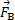are the body forces. The first term on the right-hand side of equation (1) represents the rate of change of momentum inside the control volume, while the second term represents the net flux of momentum through the control surface. The vector difference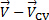is the relative velocity between the CV and the flow, and the vectoris the unit outward normal to the area differential. The dot product between the relative velocity andrepresents the velocity component that crosses the CS, and henceforth contributes to the exchange of linear momentum. The sign of this dot product is negative where the momentum flux is directed into the CV and positive where it is directed away from the CV. In this form, equation (1) is the balance of linear momentum in relation to an inertial frame of reference. Note that (1) is a vector equation, which means that in general is has three independent components. With this in mind, the analyst needs to be careful at establishing the set of forces that balance changes in linear momentum for each coordinate.

For the present demonstration, we have the configuration shown in Figure 1, where a fixed CV encloses a plate that is exposed to a plane jet. Since the jet flow is steady, there is no change of momentum inside the CV, so the first term on the right-hand side of equation (1) vanishes. Also, the CV does not move, so. Hence, the summation of forces on the CV balances with the net flux of momentum through the CS.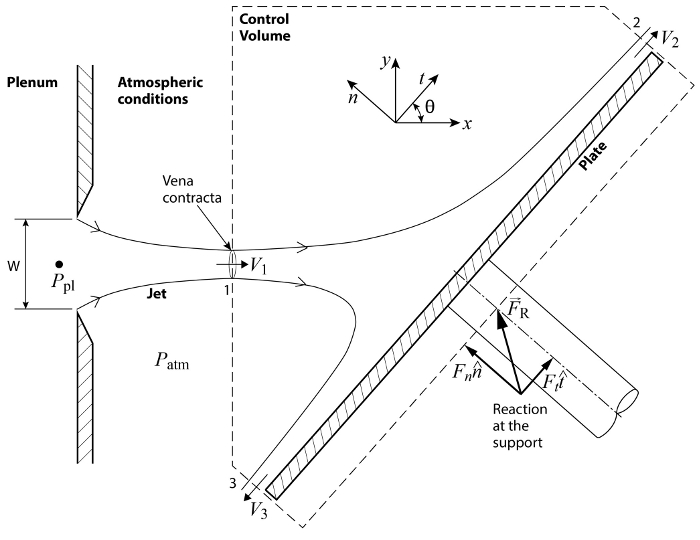Figure 1. Schematic of basic configuration. A plane jet exits the plenum through a slit of width W. The jet impinges on an inclined plate and it gets deflected while exerting a load on the surface.

Considering the configuration in Figure 1, momentum flows into the CV through port 1 and leaves the CV through ports 2 and 3. The CV crosses the incoming jet at the vena contracta, (for more information, please see video "The Interplay of Pressure and Velocity: jet impinging on an inclined plate") which is the first place at which the streamlines become parallel and, as a result, the static pressure across the jet becomes homogeneous and matches the value of the surrounding pressure, that is, atmospheric pressure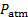. Similarly, ports 2 and 3 are located far enough from the impinging region to allow for the streamlines to become parallel and the pressure to match that of the surroundings. As a result, the pressure everywhere on the CS is equal to the atmospheric pressure,. In consequence, given that the pressure is homogeneously distributed around the CS, its net force on the control volume is zero. Additionally, since the CS was drawn perpendicular to the inlet and outlet flows, there is not any shear load induced by the flow on the CS. In summary, equation (1) simplifies to the following relationship for the case illustrated in Figure 1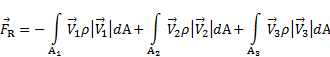(2)

Here,is the reaction of the supporting system resulting from transmission of the aerodynamic loading that the jet exerts on the plate. As shown in Figure 1, this reaction is located at the portion of the control volume that "cuts" through the supporting system of the plate. This is considered a surface force in the sense that this imaginary cut would be part of the control surface. Sinceis the only interaction with the control volume not associated with momentum flux, it is the only term on the left-hand side of equations (1) and (2). Note from comparing these equations with each other that the dot products inside the integrals result simply in the magnitudes of the corresponding velocity vectors because they are aligned with the area vectors. Also, as said before, their sign tells if the momentum flux is directed into the CV (-) or away from it (+). If we further assume that the velocity at the ports is approximately homogeneous, and that the flow is incompressible, the velocities and density can be taken outside of the integrals and equation (2) becomes: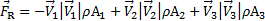(3)

Rigorously speaking, the velocity profile is never perfectly homogeneous, and this simplification requires a multiplication by a correction coefficient,, whose value depends on the details of the velocity profile. At a given flux port, this coefficient is defined as the ratio between the exact momentum flux and the momentum flux estimated from the average velocity:(4)

In turbulent flows this coefficient is very close to 1 because the velocity profile tends to be close to homogeneous. Since this is the case for the present experiment, equation (3) is a reasonable approximation for the current measurements. But if the flow rate were to be reduced or the position of the plate moved farther downstream until reaching laminar flow conditions, it would be necessary to solve the integrals on the right-hand side of equation (2) without approximation. Based on Figure 1,can be decomposed in its normal and tangent coordinates to the plate. Where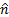andare the unit vectors in each coordinate and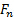and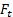are the magnitudes of the projections ofin each coordinate. Hence, equation (3) can be decomposed as: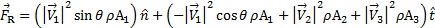(5)

Note that the minus sign on the normal component disappears because the projection ofon the normal axis is negative. We want to determine the normal load on the plate with this study because it tends to be the most relevant component from the structural point of view. From equation (4), we obtain the normal load on the plate: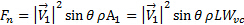(6)

Here,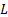is the plate span and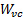is the width of the jet at the vena contracta. In general, the contraction ratio between the jet exit width,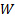, and the vena contracta is very close to [2, 3, 4]: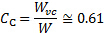(7)

In summary, the normal force on the plate can be estimated from the following relationship: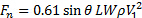(8)

Here, we define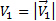for simplicity. On the other hand, the value of the term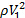is determined using Bernoulli's equation between the plenum and the vena contracta (see Figure 2 for reference). The velocity inside the plenum is considered negligible, and given that the jet is horizontal, changes in height between the plenum and the vena contracta vanish. Hence, Bernoulli's equation becomes: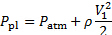(9)

Recall that the pressure at the vena contracta matches the surrounding pressure, which is atmospheric. Hence, the dynamic pressure at the vena contracta follows: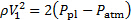(10)

Substituting equation (9) in equation (7) gives the final result to estimate the normal force on the plate based on the characteristics of the plane jet: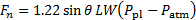(11)

This result comes from the control volume analysis of conservation of linear momentum. To have an assessment of its accuracy, we will compare these estimations to direct measurements of the force. To this end, the horizontal (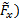and vertical (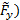components of the total force depicted in Figure 2 are captured by an aerodynamic balance. To determine the components of this measured force on the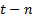coordinate system, we use the following coordinate transformation: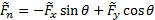(12)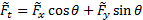(13)

Where a tilde was added to emphasize that these forces are obtained by direct measurement with an aerodynamic balance.

### Procedure

1. Setting the facility

1. Make sure that there is no flow in the facility.
2. Connect the positive port of the pressure transducer to the plenum pressure tap (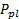).
3. Leave the negative port of the pressure transducer open to the atmosphere ().
4. Record the transducer's conversion factor from Volts to Pascals ().
5. Record the jet exit width.
6. Record the plate span.
7. Record the force balance's conversion constants from Volts to Newton (horizontal force: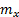; vertical force: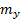).
8. Setup the data acquisition system to sample at a rate of 100 Hz for a total of 1000 samples (i.e. 10s of data).
9. Mount the impact plate on the force balance and adjust its outputs to zero.

2. Recording the data

1. Set the angle of the plate to 90o (see Figure 2 for reference).
2. Turn the flow facility on.
3. Record the reading of the pressure transducer in Volts, which corresponds to the pressure difference between the Plenum and the atmosphere (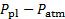).
4. Record the force data using the data acquisition system.
5. Multiply the acquired values (in Volts) by the force conversion factors (and) and enter the results in table 1.
6. Turn the flow facility off.
7. Change the angle of the plate.
8. Repeat steps 2.2 to 2.6 for the following angles: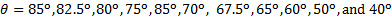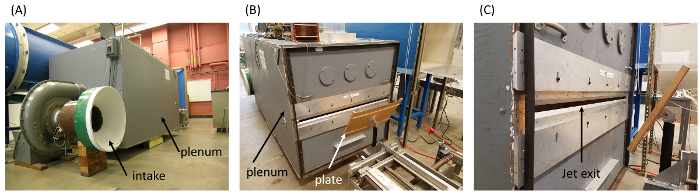Figure 2 . Experimental setting. (A): Detail of intake system to pressurize the plenum at pressure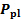. (B): discharge side with impingement plate. (C): Detail of discharge slit. Please click here to view a larger version of this figure.

3. Data Analysis

1. Calculate the normal force measured by the balance using equation(11) and record it in table 1.
2. Determine the theoretical value of the normal force from equation (10) and record it in table 1.
3. Calculate the disagreement between the two values as a percent.

Table 1 . Basic parameters for experimental study.

 Parameter Value Jet nozzle width (W) 19.05 mm Plate span (L) 110.49 cm Transducer calibration constant (m_p) 141.3829 Pa/V Balance horizontal coefficient (m_x) 22.2411 N/V Balance vertical coefficient (m_y) 4.4482 N/V

Control volume method is a powerful tool in fluid engineering, extensively used for the aerodynamic design of structures or devices. Force is developed when an object moves through a fluid. Forces exerted on bodies by a fluid flow are the result of changes in the linear momentum of the flow around them. In order to design a wind turbine blade, a boat sail, or an airplane wing, an engineer must be able to determine the dominant loads in a system. The toolbox of an engineering analyst contains methods to predict the feasibility of developing a given engineering system as well as complex methods for detailed structure calculation. This video will illustrate how to apply the control volume method to determine the aerodynamic load on a flat plate at different angles and demonstrate how loads can be estimated and measured in the laboratory.

Let's consider a plane jet impinging on a flat inclined plate. You should be familiar with this example from our previous video. Now let's take an arbitrary volume of interest around the structure named control volume, defined by an imaginary closed surface named control surface. The main principle behind control volume analysis is to replace the complex details of a system exposed to a fluid flow by a simplified free body diagram for the chosen volume. The forces acting on the system can be surface forces due to pressure or flow-induced shear. The forces acting on the system can also be body forces, for example the weight of the solids and fluids contained inside the control volume, or other forces induced by volumetric effects such as electromagnetic fields. The sum of the forces acting on the control volume equals the rate of change of the linear momentum inside the control volume and the net flux of linear momentum through the control surface, which also takes into account the speed of the control volume. This is the vector equation for the conservation of linear momentum. Now let's come back to our example and apply the principles described earlier. First, let's draw the control volume around the structure. The control volume must be chosen in ways that simplify the analysis and at the same time that capture the dominant effects on the system. Note that here the momentum flows into the control volume through port one and leaves through port two and port three. How can the equation of the momentum conservation be written for this particular configuration? Port one is placed at the location of the vena contracta where the fluid streamlines are parallel and the static pressure of the jet equals the atmospheric pressure. Assuming that ports two and three are located far enough from the impingement region, the same conditions are valid for these ports as well. Thus, the pressure is distributed homogeneously on the control surface and it is equal to atmospheric pressure. In consequence, the net pressure force acting on the control volume is zero. Since the control surface is perpendicular to the inlet and outlet flows, there is no shear load induced by the flow on the surface. The only term on the left-hand side of the equation is given by the reaction force of the plate to the transmission of the aerodynamic loading exerted by the jet on the plate. Assuming the jet flow is steady, there is no change of the momentum inside the control volume and thus the first term on the right-hand side of the equation vanishes. Since our control volume is fixed in space, the equation simplifies, showing that the reaction force to impingement equals the net flux of momentum through the control surface. The velocity vectors in our particular configuration of the control surface are aligned with the area vectors. In consequence, there is a negative influx at port one and outfluxes at ports two and three. The sum of these fluxes is the reaction force to impingement. Assuming that the velocity of the ports is approximately homogenous, the force equation simplifies further. Knowing the impingement angle theta, the resulting force can be decomposed into its normal component to the plate and its tangent component. Next, we find the normal and tangential components of the velocities at port one, port two, and respectively port three. We use these in the force equation in order to get the corresponding components of the force. The normal load on the plate is the most relevant from the structural point of view. It can also be expressed using the plate span and the width of the jet at the vena contracta. Knowing the contraction ratio between the jet exit width and the vena contracta and the dynamic pressure at the vena contracta, we obtain the final expression of the normal load on the plate estimated with the control volume analysis. In the next sections, we will measure the dominant forces exerted by an impinging jet on an inclined plate with an aerodynamic balance and then compare the measured load to the estimate based on the control volume analysis.

Before starting the experiment, check that the facility is not running. First, connect the positive port of the transducer to the pressure tap of the plenum. Leave the negative port of the pressure transducer open to sense the atmospheric pressure in the receiver. Zero the pressure transducer and record the value for the calibration constant. Set the jet exit width and measure the plate span. First, calibrate the aerodynamic balance to determine the lift conversion from volts to Newton and the drag conversion from volts to Newton. Next, record the volt to Newton conversion constants of the force aerodynamic balance device. Now record all the basic parameters of the experiment in a reference table. Next, set up the data acquisition system to capture a total of 500 samples at a rate of 100 Hertz corresponding to five seconds of data. Enter the volt to Newton conversion constants in the relevant fields of the data acquisition software. Finally, mount the impact plate on the force balance and adjust the device's outputs to zero.

To start the data acquisition, first set the angle of the plate to 90 degrees and then turn the flow facility on. First, record the reading of the pressure transducer in volts. Use this quantity together with the calibration constant from the reference table in order to calculate the pressure difference between plenum and atmosphere. Now you are ready to measure the force with the force balance. To do this, use the data acquisition system to record force data. The data acquisition system will automatically use the conversion factors to determine force using the measurements in volts. Enter the results in a table. Turn the flow facility off and change the angle of the plate. Next, turn on the flow facility and repeat the force measurements for different angles. Record the data in a results table.

Calculate the normal force exerted on a flat plate by using the angle theta and the experimental values for the horizontal and the vertical components of the impingement force measured with the aerodynamic balance. Repeat the calculation for each angle theta and record the values in the results table. Using the parameters table and the measured values of the pressure difference between plenum and atmosphere, calculate the theoretical value of the normal impingement force on the plate. Repeat the calculation for each angle theta and record the values in the results table. Calculate the disagreement between the measured and theoretical values of the impingement force. Repeat the calculation for each angle theta and record the values in the results table.

Begin by plotting the load on the plate given by direct measurements with an aerodynamic balance as a function of the impingement angle theta. Place on the same graph the load calculated using the theoretical analysis with the control volume approach together with the percent error epsilon. Now compare the values directly measured with the values calculated with the control volume analysis for each load exerted on the plate at each angle theta. The discrepancies between the two methods vary non-monotonically with the angle theta and range between 2% and 12.5%. For angles smaller than and equal to 80 degrees, the control volume method underestimated the loads on the plate. While for angles higher than 80 degrees, this method gave values higher than the measured loads. The differences could be due to the fact that the control volume analysis assumes inviscid non-dissipative changes in linear momentum. While the direct measurements cannot avoid the effect of viscosity on the flow.

Control volume analysis of the conservation of linear momentum is widely used to predict the feasibility of developing a given engineering system before pursuing a detailed aerodynamic design of the structure or device. A Pelton blade is designed to convert the highest amount of linear momentum into torque. Control volume analysis has demonstrated that the blade geometry that maximizes the change in linear momentum of water jets is such that imposes a change of direction of 180 degrees in jet trajectory. In order to predict the effects of the wind on a real-sized structure, experiments can be conducted with a downscaled model in wind or water tunnels. Here the control volume analysis is used together with velocity measurements upstream and downstream of the model in order to determine the effective load of the prototype.

You've just watched Jove's introduction to the control volume analysis of the conservation of linear momentum. You should now understand the basic principles of the method and how to apply them to estimate forces exerted by flow on a structure. You have also learned how to perform force measurements with an aerodynamic balance. Thanks for watching.

### Results

Figure 3 shows a comparison between the normal load on the flat plate as measured directly from an aerodynamic balance and estimated from conservation of linear momentum. In general, the analysis of linear momentum captured the dominant tendency of direct measurements as the impingement angle changes. The discrepancies in these measurements varied non-monotonically with the impingement angle. For impingement angles in the range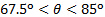, and for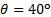, discrepancies are below 6%. They are higher for the other angles, but never higher than 12.5%. There appears to be a crossover around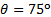, in which the tendency of discrepancies invert: measurements exhibit higher normal loads than analysis of linear momentum for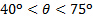and lower for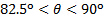. These differences in the tendencies could be due to the fact that the analysis of linear momentum assumes inviscid, non-dissipative, changes in linear momentum, while direct measurements cannot avoid the effect of viscosity on the flow. For the range, the shear component becomes dominant and therefore turbulent boundary layer effects could be important. In this case, wall-normal velocity fluctuations due to turbulence might be responsible for the increase in the normal load. On the other hand, the axial velocity of the jet experiences a significant reduction in the rangewhile it turns to become dominantly tangent to the wall. This effect is likely to let viscosity dissipate viscosity due to a reduction in the local values of Reynolds number, and that would result in reduced values of the normal load.

Table 2. Representative results.

 θ F ̃_x(N) F ̃_y (N) F ̃_n (N) F_n (N) ε (%) 90o 15.257 9.034 15.257 16.773 9.9 85o 15.151 9.831 15.950 16.709 4.8 82.5o 15.035 10.231 16.242 16.630 2.4 80o 15.929 10.498 17.510 16.518 5.7 75o 14.248 10.453 16.468 16.202 1.6 70o 13.518 11.405 16.604 15.762 5.1 67.5o 13.100 11.294 16.425 15.496 5.7 65o 12.771 11.579 16.468 15.202 7.7 60o 11.881 11.863 16.221 14.526 10.5 50o 9.746 11.241 14.691 12.849 12.5 40o 6.357 9.444 11.320 10.782 4.8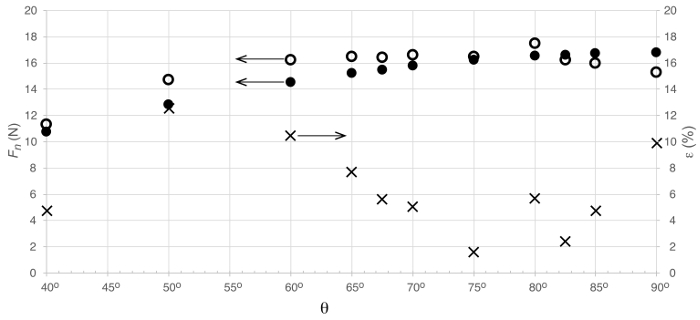Figure 3. Representative results. Load on plate as a result of impinging jet. Symbols represent: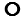: direct load measurement;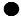: estimation from conservation of linear momentum;: percent error between experimental measurements and theoretical estimation.

### Applications and Summary

We demonstrated the application of control volume analysis of conservation of linear momentum to determine the forces exerted by a jet impinging on a flat plate. This analysis proved simple to apply, and gave a satisfactory bulk estimation of loads without requiring detailed knowledge of the flow pattern around the plate. Though there were some discrepancies (both in magnitude as well as tendency) due to the basic assumption of inviscid transformation of momentum, this technique offers a means of obtaining a quick estimation of system behavior without delving into a detailed study of fluid flow. Hence, this is a powerful tool for the engineering analyst to, for instance, predict the feasibility of developing a given engineering system with a minimal investment of time and resources. Once this first analysis is conducted to determine feasibility, the engineer can move into a more detailed flow analysis using, for example, computational fluid dynamics.

Control volume analysis of conservation of linear momentum is a powerful tool for fluids engineering. It finds application in a wide variety of problems to circumvent more involved methods such as differential analysis. A few instances of this analysis can be described:

Pelton turbine blade design: in general, a Pelton turbine blade should be designed to convert the highest amount of linear momentum into torque. This is achieved by determining the geometry of the blade that maximizes the change in the linear momentum of water jets. To this end, the typical result of control volume analysis is that the jet should be made to turn around on itself, that is, 180o. This is in general a technical challenge for a rotating device, but gives the analyst an initial guidance for a more detailed analysis using other tools.

Drag load on civil structures: one of the challenges of civil engineering is to design structures that stand the load of wind. In order to predict the effects of the wind on a real-size structure, it is possible to conduct experiments with a down-scaled model in wind or water tunnels. To this end, it is possible to use control volume analysis of conservation of linear momentum based on velocity measurements upstream and downstream of the model to determine the effective load on the prototype. This method both simplifies the experimental campaign and saves time, effort, and money in preparation for the construction of a real-scale structure.X